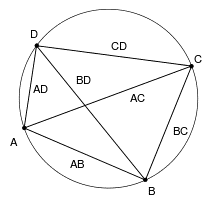## PtolemyClaudius Ptolemaeus was a mathematician, astronomer, geographer and astrologer who wrote several scientific treatises. The first is the astronomical treatise now known as the Almagest. The second is the Geography, which is a thorough discussion of the geographic knowledge of the Greco-Roman world. The third is the astrological treatise in which he attempted to adapt horoscopic astrology to the Aristotelian natural philosophy of his day.

Ptolemy’s Theorem

Ptolemy’s theorem is a relation between the four sides and two diagonals of a cyclic quadrilateral (a quadrilateral whose vertices lie on a common circle). Ptolemy used the theorem as an aid to creating his table of chords, a trigonometric table that he applied to astronomy.If the vertices of the cyclic quadrilateral are A, B, C, and D in order, then the theorem states that: